Introduction to Fixed Income Valuation | IFT World
IFT Notes for Level I CFA® Program
IFT Notes for Level I CFA® Program

# Part 1

## 1.  Introduction

In this reading, we will see how to value different types of fixed-income securities such as fixed-rate bonds, floating-rate bonds, and money market securities. This reading will cover:

• How to price a bond.
• How bond prices and yields are calculated.
• The term structure of interest rates.
• Yield spreads over benchmark interest rates.

## 2.  Bond Prices and the Time Value of Money

### 2.1.     Bond Pricing with a Market Discount Rate

A bond’s price is the present value of all future cash flows at the market discount rate. The discount rate is the rate of return required by investors given the risk of investment in the bond. It is also known as the required yield, or required rate of return.

PV of bond =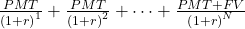where:
PMT = coupon payment per period
FV= par value of the bond paid at maturity
r = market discount rate
N = number of periods until maturity

Example

The coupon rate on a bond is 4% and the payment is made once a year. The time to maturity is five years and the market discount rate is 6%. What is the bond price per 100 of par value?

Solution:

Start by drawing a timeline for the cash flows. The par value of the bond or principal is $100. A coupon payment of$4 is made every year. At maturity (at the end of five years), a payment of $104 (principal of$100 + coupon of $4) is made. We are required to calculate the present value of bond at time t = 0. For that, we discount all the future cash flows at the market discount rate of 6%.  End of year Type of cash flow Amount Present value 1 Coupon 4 3.77 2 Coupon 4 3.55 3 Coupon 4 3.36 4 Coupon 4 3.17 5 Coupon + principal 104 77.71 91.56 Using the formula, PV =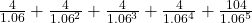= 91.575 Note: The table and formula above were just for your understanding. On the exam, solve this quickly using a financial calculator: N = 5; I/Y = 6; FV = 100; PMT = 4; PV = ? PV = -91.575 Notice the present value of the bond (91.575) is lower than the par value of the bond (100). Premium, Par and Discount Bonds Example Assume we have another bond with a coupon rate of 8%, paid annually. If the market discount rate is again 6%, what is the price of the bond? Solution: Note that the coupon rate here is higher than the market rate. Calculate PV using these input values: N = 5; I/Y = 6; PMT = 8; FV = 100; CPT PV PV = -108.425. In this case, the present value of the bond is higher than its par value. Such a bond is called a premium bond. Since the coupon rate (8%) is higher than the required return of 6%, investors pay more than the par value for the bond. What if the coupon rate is 6%? N = 5; I/Y = 6; PMT = 6; FV = 100; CPT PV PV = -100. The present value is equal to the par value of the bond. What if the coupon rate is 2%? N = 5; I/Y = 6; PMT = 2; FV = 100; CPT PV PV = -83.151. This type of bond, which sells at a price below its par value, is called a discount bond. Investors require a return of 6% but it pays a coupon of only 2%, so investors pay less.  Par, premium or discount Relationship between coupon rate & market discount rate Par bond Coupon rate = market discount rate Discount bond Coupon rate < market discount rate Premium bond Coupon rate > market discount rate ### 2.2. Yield to maturity The yield to maturity (YTM) is the internal rate of return on the cash flows – the uniform interest rate that will make the sum of the present values of future cash flows equal to the price of the bond. It is the implied market discount rate. In simpler terms, it is a bond’s internal rate of return – the rate of return on a bond including interest payments and capital gain if the bond is held until maturity. Yield to maturity is based on three important assumptions: • The investor holds the bond to maturity. • The issuer does not default on payments and pays coupon and principal as they come due. • The investor is able to reinvest all proceeds (coupons) at the YTM. (This is an unrealistic assumption as the interest rates may increase or decrease after the bond is purchased. So the coupon may be reinvested at a higher or lower rate.) Example A$100 face value bond with a coupon rate of 10% has a maturity of 4 years. The price of the bond is \$80. What is its yield to maturity?

Solution:

Based on YTM’s definition, we come up with the equation below: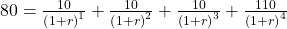This equation can be solved using trial and error but it is much easier to use a financial calculator: N = 4; PMT = 10; FV = 100; PV= -80; CPT I/Y = 17.34%. The bond is trading at a discount. So the coupon rate (10%) must be lower than market rate (17.34%).

Example

Calculate the yields-to-maturity for the following bonds:

 Bond Coupon Payment per Period Number of Periods to Maturity Price A 2.50 3 102.80 B 2.00 5 97.76 C 0.00 48 23.425

Solution:

Bond A: 102.80 =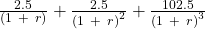,

r = 1.54%

Bond B: 97.76 =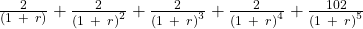,

r = 2.48%

Bond C: 23.425 =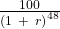, r = 3.07%

Zoom Classes for Nov-23 Exam Starting Now!
This is default text for notification bar